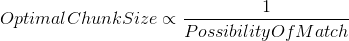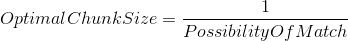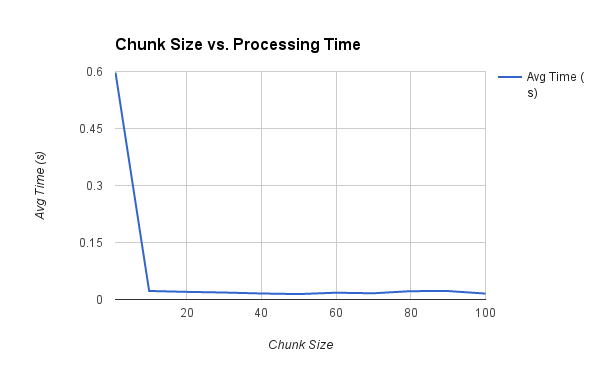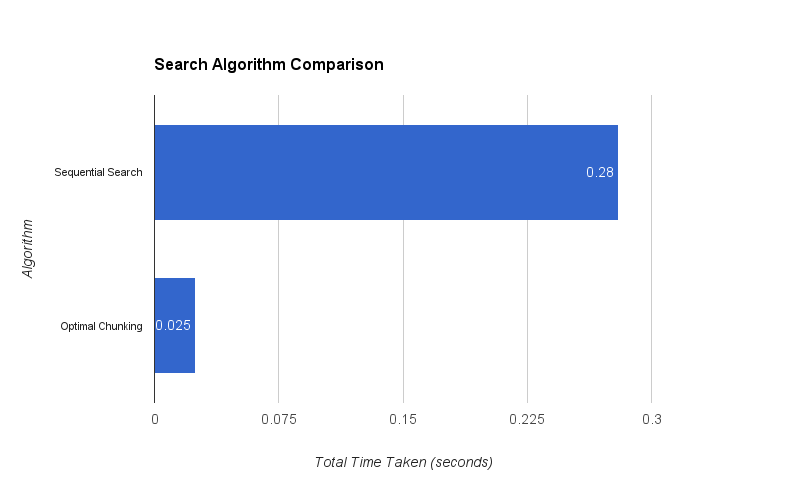A large part of my job involves natural language processing. What I repeatedly find myself doing is searching through large lists of regular expressions, and doing something based on the expressions that have been matched.

This is problematic, as there aren’t any data structures/algorithms for efficient regular expression searching in this manner, and you cannot combine multiple regular expressions into a single search as you need to know which expressions have been matched. Previously, I had been using sequential search. While sequential search allows you to see which expressions have been matched, its performance is poor. The NLP system I maintain needs to be able to process large amounts of data in near real time. As such, a better algorithm was needed.

## Sequential Search Algorithm

To start, I first analysed the sequential search algorithm I was using, as any performance gains would be measured relative to it.

### Sequential Search Pseudocode### Sequential Search Time Complexity

Best Case Worst Case
O(n) O(n)
• n: number of regular expressions in the list

As shown in the time complexity table above, the time complexity in the best and worst cases is the same. While a linear worst case time complexity is fair for a search algorithm, a linear best case time complexity is quite bad.

## Improved Search Algorithm

The improved algorithm works by splitting the lists of regular expressions up into chunks, and doing a single preliminary regular expression search for each chunk. If the preliminary search finds a match, then it will search for each regular expresion in the chunk individually.

### Chunking Algorithm Pseudocode### Chunking Algorithm Time Complexity

Best Case Worst Case
O(c) O(cn)
• c: number of chunks
• n: number of regular expressions in a chunk

The improved classification algorithm has a best case that is far better than the old classification algorithm, however it also has a worst case which is worse. For example, take a list of 1000 words and a chunk size of 100. The old classification algorithm would iterate 1000 times in the best and worst case, whereas the new classification algorithm would iterate 10 times in the best case and 1010 times in the worst. This potential 1% increase in the number of worst case iterations is far out weighed by the potential 99% reduction in the number of best case iterations, although this will vary based on the chunk size chosen.

It is also highly unlikely that the worst case will even occur. This is because for the worst case to occur the number of matches found needs to be greater than or equal to the number of chunks. For example, in the case above the text would need to have ten matches. The natural language processing I do is focused on micro-blogging sites such as Twitter. Twitter limits tweets to 140 characters, which effectively limits the number of words in a tweet. This means that, if an appropriate chunk size is chosen, the worst case is unlikely to occur.

## Optimal Chunk Size

Optimal chunk size is inversely proportional to the probability of a match. Once the probability of a match has been found, optimal chunk size can be calculated.To test the relationship between chunk size and processing time, I recorded processing time for a range of chunk sizes. I searched against text that had an equal probability of 0 - 3 matches. The graph below shows the results of this test.As the graph shows, chunk size can have a dramatic effect on processing time.

Once I had calculated the probability of a match, I used the formula above to calculate the optimal chunk size for my application. With an appropriate chunk size chosen, the chunking algorithm has reduced processing time by ~90% compared to sequential search, effectively solving the performance issues we were having.